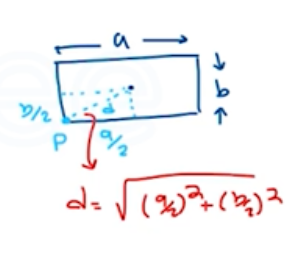Need Help?

Subscribe to Physics 1

###### \${selected_topic_name}
• Notes

$\begin{array}{l}{\text { A compound disk of outside diameter } 140.0 \mathrm{cm} \text { is made }} \\ {\text { up of a uniform solid disk of radius } 50.0 \mathrm{cm} \text { and area density }} \\ {3.00 \mathrm{g} / \mathrm{cm}^{2} \text { surrounded by a concentric ring of inner radius } 50.0 \mathrm{cm},} \\ {\text { outer radius } 70.0 \mathrm{cm}, \text { and area density } 2.00 \mathrm{g} / \mathrm{cm}^{2} . \text { Find the }} \\ {\text { moment of inertia of this object about an axis perpendicular to the }} \\ {\text { plane of the object and passing through its center. }}\end{array}$

$r_{1}=50 \mathrm{cm}$

$r _2=70 \mathrm{cm}$

$\begin{array}{c}{\text { Solid desk }} \\ {\downarrow}\end{array}$

$I_{d}=\frac{1}{2} m_{d} {r_{d}}^{2}$

$\begin{array}{c}{\text { Ring }} \\ {\downarrow}\end{array}$

$I_{r}=\frac{1}{2} m_{r}\left({r_{1}}^{2}+{r_{2}}^{2}\right)$

$\rightarrow I=I_{d}+I_{r}$

$m_{d}=\left(3 \text { g/cm }^{2}\right) \pi {r_{d}}^{2}=23.56 \mathrm{kg}$

$∴ I_{d}=\frac{1}{2}(23.56)\left(\frac{(1.4)}{2}\right)^{2}=2.945 \ kg \cdot m^{2}$

$m _r=(2 g/c m^{2}) \pi ({r_{2}}^{2}-{r_{1}}^{2})=15.08 \mathrm{kg}$

$∴ I_{r}=\frac{1}{2}(15.08)(0.5^2+0.7^2)=5.58 kg.m^2$

$I=2.945+5.58$

$=8.52 \mathrm{kg.m}^{2}$

$\begin{array}{l}{\text { A uniform sphere with mass } 28.0 \mathrm{kg} \text { and radius } 0.380 \mathrm{m} \text { is }} \\ {\text { rotating at constant angular velocity about a stationary axis that }} \\ {\text { lies along a diameter of the sphere. If the kinetic energy of the }} \\ {\text { sphere is } 176 \mathrm{J} \text { , what is the tangential velocity of a point on the rim }} \\ {\text { of the sphere? }}\end{array}$

$K=\frac{1}{2} I \omega^{2} \rightarrow I=\frac{2}{5} M R^{2}$

$I=\frac{2}{5}(28)(0.38)^{2}=1.617 \mathrm{kg} \cdot \mathrm{m}^{2}$

$k=\frac{1}{2}(1.617) \omega^{2} \longrightarrow \omega=\sqrt{\frac{2 k}{I}}=\sqrt{\frac{2(176)}{1.617}}$

$∴ \omega=14.75 \text { rad } / \mathrm{s}$

$\omega=\frac{v}{r} \rightarrow v=\omega. r$

$V=(14.75)(0.38)=5.61 \mathrm{m} / \mathrm{s}$

$\begin{array}{l}{\text { A thin, rectangular sheet of metal has mass } M \text { and sides }} \\ {\text { of length } a \text { and } b . \text { Use the parallel-axis theorem to calculate the }} \\ {\text { moment of inertia of the sheet for an axis that is perpendicular to the }} \\ {\text { plane of the sheet and that passes through one corner of the sheet. }}\end{array}$

$I_p=I_{c m}+M d^{2}$$(9 \cdot 2) \rightarrow(c)$

$I_{c m}=\frac{1}{12} M\left(a^{2}+b^{2}\right)$

$\rightarrow I_p= I_{c m}+M d^{2}$

$=\frac{1}{12} M\left(a^{2}+b^{2}\right)+M\left(\frac{a^{2}}{4}+\frac{b^{2}}{4}\right)$

$=\left(\frac{1}{12}+\frac{1}{4}\right) M\left(a^{2}+b^{2}\right)=\left(\frac{1}{4}+\frac{1}{12}\right) M\left(a^{2}+b^{2}\right)$

$∴ I_{P}=4 \ I_{c m}$